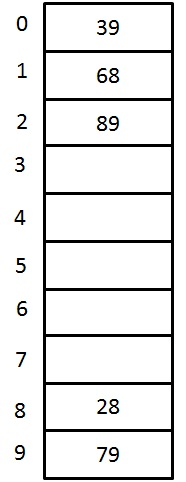# Hashing Collision Resolution - Linear Probing

## Linear Probing

Linear Probing is one of the 3 open addressing / closed hashing collision resolution techniques

This is a simple method, sequentially tries the new location until an empty location is found in the table.
For example: inserting the keys {79, 28, 39, 68, 89} into closed hash table by using same function and collision resolution technique as mentioned before and the table size is 10 ( for easy undestanding we are not using prime number for table size). The hash function is hi(X) = ( Hash(X) + F(i)) % TableSize for i = 0, 1, 2, 3,...etc.
Solution:Figure 3. A Closed Hash Table using Linear Probing

Key   Hash Function h(X)         IndexCollisionAlt Index
79  h0(79)
= (Hash(79)+F(0))%10
= ((79%10)+0)%10    = 9
9
28  h0(28)
= (Hash(28)+F(0))%10
= ((28%10)+0)%10     = 8
8
39  h0(39)
= (Hash(39)+F(0))%10
= ((39%10)+0)%10     = 9
9  first
collision
occurs
h1(39)
= (Hash(39)+F(1))%10
= ((39%10)+1)%10     = 0
0 0
68  h0(68)
= (Hash(68)+F(0))%10
= ((68%10)+0)%10     = 8
8 collision
occurs
h1(68)
= (Hash(68)+F(1))%10
= ((68%10)+1)%10     = 9
9 Again
collision
occurs
h2(68)
= (Hash(68)+F(2))%10
= ((68%10)+2)%10     = 0
0 Again
collision
occurs
h3(68)
= (Hash(68)+F(3))%10
= ((68%10)+3)%10     = 1
1 1
89  h0(89)
= (Hash(89)+F(0))%10
= ((89%10)+0)%10     = 9
9 collision
occurs
h1(89)
= (Hash(89)+F(1))%10
= ((89%10)+1)%10     = 0
0 Again
collision
occurs
h2(89)
= (Hash(89)+F(2))%10
= ((89%10)+2)%10     = 1
1 Again
collision
occurs
h3(89)
= (Hash(89)+F(3))%10
= ((89%10)+3)%10     = 2
2 2

The problem with linear probing is primary clustering. This means that even if the table is empty, any key that hashes to table requires several attempt to resolve the collision because it has to cross over the blocks of occupied cell. These blocks of occupied cell form the primary clustering. If any key falls into clustering, then we cannot predict the number of attempts needed to resolve the collision. These long paths affect the performance of the hash table.

# Hashing Collision Resolution - Linear Probing

## Linear Probing

Linear Probing is one of the 3 open addressing / closed hashing collision resolution techniques

This is a simple method, sequentially tries the new location until an empty location is found in the table.
For example: inserting the keys {79, 28, 39, 68, 89} into closed hash table by using same function and collision resolution technique as mentioned before and the table size is 10 ( for easy undestanding we are not using prime number for table size). The hash function is hi(X) = ( Hash(X) + F(i)) % TableSize for i = 0, 1, 2, 3,...etc.
Solution:Figure 3. A Closed Hash Table using Linear Probing

Key   Hash Function h(X)         IndexCollisionAlt Index
79  h0(79)
= (Hash(79)+F(0))%10
= ((79%10)+0)%10    = 9
9
28  h0(28)
= (Hash(28)+F(0))%10
= ((28%10)+0)%10     = 8
8
39  h0(39)
= (Hash(39)+F(0))%10
= ((39%10)+0)%10     = 9
9  first
collision
occurs
h1(39)
= (Hash(39)+F(1))%10
= ((39%10)+1)%10     = 0
0 0
68  h0(68)
= (Hash(68)+F(0))%10
= ((68%10)+0)%10     = 8
8 collision
occurs
h1(68)
= (Hash(68)+F(1))%10
= ((68%10)+1)%10     = 9
9 Again
collision
occurs
h2(68)
= (Hash(68)+F(2))%10
= ((68%10)+2)%10     = 0
0 Again
collision
occurs
h3(68)
= (Hash(68)+F(3))%10
= ((68%10)+3)%10     = 1
1 1
89  h0(89)
= (Hash(89)+F(0))%10
= ((89%10)+0)%10     = 9
9 collision
occurs
h1(89)
= (Hash(89)+F(1))%10
= ((89%10)+1)%10     = 0
0 Again
collision
occurs
h2(89)
= (Hash(89)+F(2))%10
= ((89%10)+2)%10     = 1
1 Again
collision
occurs
h3(89)
= (Hash(89)+F(3))%10
= ((89%10)+3)%10     = 2
2 2

The problem with linear probing is primary clustering. This means that even if the table is empty, any key that hashes to table requires several attempt to resolve the collision because it has to cross over the blocks of occupied cell. These blocks of occupied cell form the primary clustering. If any key falls into clustering, then we cannot predict the number of attempts needed to resolve the collision. These long paths affect the performance of the hash table.